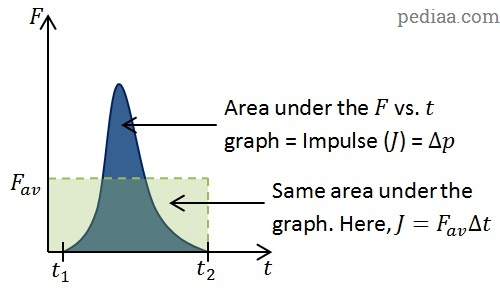# What is Linear Momentum

Linear Momentum ($\vec{p}$) of a body is defined as the product of the body’s mass and velocity.$\vec{p}=m\vec{v}$

Linear momentum is a vector quantity, having both a magnitude and a direction. The direction of the momentum vector is in the direction of the body’s velocity. The SI unit for measuring momentum is kg m s-1.

Momentum is an extremely useful quantity to calculate, as it is conserved in closed systems.

## Rate of Change of Momentum

In order to change a body’s momentum, a force must be applied to it. The net force required is equal to the rate of change of momentum. In symbols, we can write as:$\sum \vec{F}=\frac{\mathrm{d} \vec{p}}{\mathrm{d}t}$

This is a statement of Newton’s second law of motion. In fact, this is closer to the form that Newton himself used to express the law in. As we have seen in our discussion of Newton’s second law when the mass of the body stays constant, we can use this equation to recover the more familiar expression of Newton’s second law,$\sum \vec{F}=m\vec{a}$.

For considering cases where the mass of a body changes (for rockets, for example), we come up with another expression.$\sum \vec{F}=\frac{\mathrm{d} \vec{p}}{\mathrm{d}t}=\frac{\mathrm{d}\left( m\vec{v}\right)}{\mathrm{d}t}$. Using the chain rule, we get:$\sum \vec{F}=m\frac{\mathrm{d}\vec{v}}{\mathrm{d}t}+\vec{v}\frac{\mathrm{d}m}{\mathrm{d}t}$

## Impulse | Impulse-Momentum Theorem

Let us consider the collision between two objects. e.g. the collision between the tennis racket and the ball when a player serves. For a spectator, the collision seems instantaneous, but this is not the case. If you used a high-speed camera, recorded a tennis serve and then slowed it down, you would notice that the racket and the ball are in contact for some time, during which both the racket and the ball get deformed. During this time, the force that the racket exerts on the ball is not constant.What is Linear Momentum – A Tennis Serve

Let us assume that the racket and the ball first came to contact at a time$t=t_1$ and that the contact lasted until a time$t=t_2$. Taking the equation$\vec{F}=\frac{\mathrm{d} \vec{p}}{\mathrm{d}t}$, we can rearrange and integrate over the time period to obtain the total force:$\int_{t_1}^{t_2}\vec{F}\mathrm{d}t=\int_{t_1}^{t_2}\mathrm{d}\vec{p}$

If we take the change in momentum to be$p_2-p_1=\Delta \vec{p}$, we can write$\int_{t_1}^{t_2}\vec{F}\mathrm{d}t=\Delta \vec{p}$

The quantity$\int_{t_1}^{t_2}\vec{F}\mathrm{d}t$ is the area under a force vs. time graph. It is also called impulse ($\vec{J}$):$\int_{t_1}^{t_2}\vec{F}\mathrm{d}t=\vec{J}$

and, as we saw above,$\vec{J}=\Delta \vec{p}$

This above expression is sometimes referred to as the impulse-momentum theorem.

Units of impulse are kg m s-1 or N s.

If we draw a graph of how the force acting between two bodies in a collision varies over time, we would get the blue curve on the following graph. As we mentioned before, the area under this graph is equal to the impulse. Note that we can come up with an average force, ($F_{av}$), such that$F_{av}\Delta t=J$.What is Linear Momentum – Force vs Time Graph

## Linear Momentum Example

### Force Exerted on a Wall by Water from a Hose Pipe

Suppose a water pipe with cross-sectional area$A$ carrying water at a speed$v$ is aimed horizontally at a wall. We can find the force$F$ exerted on the wall by the water from the pipe:What is Linear Momentum – Force on a wall by water from a horizontal hosepipe$F=\frac{\Delta p}{\Delta t}=\frac{m\Delta v}{\Delta t}=\frac{m}{\Delta t}\times\Delta v$$\Delta v=$ change in velocity of water. Once the water hits the wall, it travels down the wall, losing all horizontal velocity. Therefore,$\Delta v=v_f-v_i=0-v=-v$.$\frac{m}{\Delta t}=$ mass of water per second (the flow rate)$=\frac{\rho V}{\Delta t}$, where$\rho$ is the density of water and$V$ is the volume. Now,$\frac{V}{\Delta t}=$ volume of water exiting the pipe per second. Since cross-sectional area is$A$$\frac{V}{\Delta t}=\frac{\rho Al}{\Delta t}$, where$l$ is the distance travelled by water per second.

Now, we have$F=\frac{\rho Al}{\Delta t}\times\left(-v\right)$. Since$\frac{l}{\Delta t}=v$, we have:$F=-\rho Av^2$

The negative sign indicates that the force exerted on water by the wall is to the left (in this diagram). The force exerted by the water on the wall must have the same magnitude but act in the opposite direction (according to Newton’s third law). So, force exerted by the water on the wall is:$F=\rho Av^2$

Example 1

A tennis ball having a mass of 0.058 kg is thrown upwards in the air and struck horizontally with a racket. After being in contact with the racket for 0.01 s, the ball leaves with a horizontal velocity of 54 m s-1. Calculate the average force exerted on the ball.$F=\frac{m\Delta v}{\Delta t}=\frac{0.058\times\left(54-0\right)}{0.01}=310\mathrm{\: N}$.

###### “serve of girl at tennis” by Jeuwre (Own work) [CC BY-SA 4.0], via Wikimedia Commons